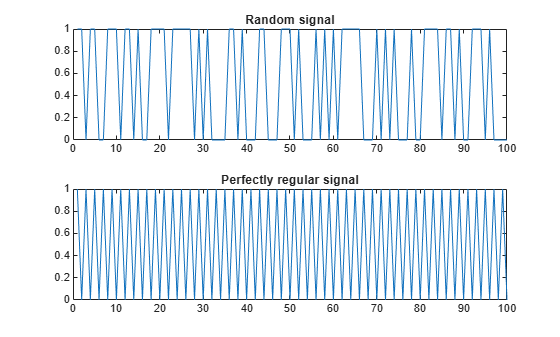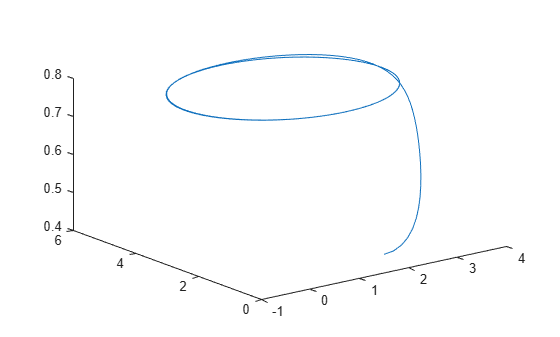# approximateEntropy

Measure of regularity of nonlinear time series

## Syntax

``approxEnt = approximateEntropy(X)``
``approxEnt = approximateEntropy(X,lag)``
``approxEnt = approximateEntropy(X,[],dim)``
``approxEnt = approximateEntropy(X,lag,dim)``
``approxEnt = approximateEntropy(___,Name,Value)``

## Description

example

````approxEnt = approximateEntropy(X)` estimates the approximate entropy of the uniformly sampled time-domain signal `X` by reconstructing the phase space. Approximate entropy is a measure to quantify the amount of regularity and unpredictability of fluctuations over a time series.```

example

````approxEnt = approximateEntropy(X,lag)` estimates the approximate entropy for the time delay `lag`.```

example

````approxEnt = approximateEntropy(X,[],dim)` estimates the approximate entropy for the embedding dimension `dim`.```

example

````approxEnt = approximateEntropy(X,lag,dim)` estimates the approximate entropy for the time delay `lag` and the embedding dimension `dim`.```

example

````approxEnt = approximateEntropy(___,Name,Value)` estimates the approximate entropy with additional options specified by one or more `Name,Value` pair arguments.```

## Examples

collapse all

For this example, generate two signals for comparison $-$ a random signal `xRand` and a perfectly regular signal `xReg`. Set `rng` to `default` for reproducibility of the random signal.

```rng('default'); xRand = double(randn(100,1)>0); xReg = repmat([1;0],50,1);```

Visualize the random and regular signals.

```figure; subplot(2,1,1); plot(xRand); title('Random signal'); subplot(2,1,2); plot(xReg); title('Perfectly regular signal');```The plots show that the regular signal is more predictable than the random signal.

Find approximate entropy of the two signals.

`valueReg = approximateEntropy(xReg)`
```valueReg = 5.1016e-05 ```
`valueIrreg = approximateEntropy(xRand)`
```valueIrreg = 0.6849 ```

The approximate entropy of the perfectly regular signal is significantly smaller than the random signal. Hence, the perfectly regular signal containing many repetitive patterns has a relatively small value of approximate entropy while the less predictable random signal has a higher value of approximate entropy.

In this example, consider the position data of a quadcopter, following a circular path. The file `uavPositionData.mat` contains the x, y and z-direction position data traversed by the copter.

Load the data set and visualize the quadcopter path in 3D.

```load('uavPositionData.mat','xv','yv','zv'); plot3(xv,yv,zv);```For this example, use only x-direction position data for computation. Since `Lag` is unknown, estimate the delay using `phaseSpaceReconstruction`. Set `'Dimension'` to 3. The `Dimension` and `Lag` parameters are required to compute the approximate entropy of the data.

```dim = 3; [~,lag] = phaseSpaceReconstruction(xv,[],dim)```
```lag = 4 ```

Find the approximate entropy using the `Lag` value obtained in the previous step.

`approxEnt = approximateEntropy(xv,lag,dim)`
```approxEnt = 0.1088 ```

Since the quadcopter is traversing a pre-defined circular trajectory of fixed radius, the position data is regular and hence, the value of approximate entropy is low.

## Input Arguments

collapse all

Uniformly sampled time-domain signal, specified as either a vector, array, or timetable. If `X` has multiple columns, `approximateEntropy` computes the approximate entropy by treating `X` as a multivariate signal.

If `X` is specified as a row vector, `approximateEntropy` treats it as a univariate signal.

Embedding dimension, specified as a scalar or vector. `dim` is equivalent to the '`Dimension`' name-value pair.

Time delay, specified as a scalar or vector. `lag` is equivalent to the '`Lag`' name-value pair.

### Name-Value Arguments

Specify optional pairs of arguments as `Name1=Value1,...,NameN=ValueN`, where `Name` is the argument name and `Value` is the corresponding value. Name-value arguments must appear after other arguments, but the order of the pairs does not matter.

Before R2021a, use commas to separate each name and value, and enclose `Name` in quotes.

Example: `...,'Dimension',3`

Embedding dimension, specified as the comma-separated pair consisting of '`Dimension`' and a scalar or vector. When `Dimension` is scalar, every column in `X` is reconstructed using `Dimension`. When `Dimension` is a vector having same length as the number of columns in `X`, the reconstruction dimension for column `i` is `Dimension(i)`.

Specify `Dimension` based on the dimension of your system. For more information on embedding dimension, see `phaseSpaceReconstruction`.

Delay in phase space reconstruction, specified as the comma-separated pair consisting of '`Lag`' and a scalar. When `Lag` is scalar, every column in `X` is reconstructed using `Lag`. When `Lag` is a vector having same length as the number of columns in `X`, the reconstruction delay for column `i` is `Lag(i)`.

If the delay is too small, random noise is introduced in the data. In contrast, if the lag is too large, the reconstructed dynamics does not represent the true dynamics of the time series. For more information on calculating optimal delay, see `phaseSpaceReconstruction`.

Similarity criterion, specified as the comma-separated pair consisting of '`Radius`' and a scalar. The similarity criterion, also called radius of similarity, is a tuning parameter that is used to identify a meaningful range in which fluctuations in data are to be considered similar.

The default value of `Radius` is,

• `0.2*std(X)`, if `X` has a single column.

• `0.2*sqrt(trace(cov(X)))`, if `X` has multiple columns.

## Output Arguments

collapse all

Approximate entropy of nonlinear time series, returned as a scalar. Approximate entropy is a regularity statistic that quantifies the unpredictability of fluctuations in a time series. A relatively higher value of approximate entropy reflects the likelihood that similar patterns of observations are not followed by additional similar observations.

For example, consider two binary signals `S1` and `S2`,

S1 = [0 1 0 1 0 1 0 1 0 1 0 1 0 1 0 1];

S2 = [1 1 0 1 1 1 1 0 1 0 1 0 0 0 0 1];

Signal `S1` is perfectly regular since it alternates between `0` and `1`, that is, you can predict the next value with knowledge of the previous value. Signal `S2` however offers no insight into the next value, even with prior knowledge of the previous value. Hence, signal `S2` is random and less predictable. Therefore, a signal containing highly repetitive patterns has a relatively small value of `approxEnt` while a less predictable signal has a relatively larger value of `approxEnt`.

Use `approximateEntropy` as a measure of regularity to quantify levels of complexity within a time series. The ability to discern levels of complexity within data sets is useful in the field of engineering to estimate component failure by studying their vibration and acoustic signals, or in the clinical domain where, for instance, the chance of a seizure is predicted by observing Electroencephalography (EEG) patterns.

## Algorithms

Approximate entropy is computed in the following way,

1. The `approximateEntropy` function first generates a delayed reconstruction Y1:N for N data points with embedding dimension m, and lag τ.

2. The software then calculates the number of within range points, at point i, given by,

`${N}_{i}=\sum _{i=1,i\ne k}^{N}1\left({‖{Y}_{i}-{Y}_{k}‖}_{\infty }`

where 1 is the indicator function, and R is the radius of similarity.

3. The approximate entropy is then calculated as $approxEnt={\Phi }_{m}-{\Phi }_{m+1}$ where,

`${\Phi }_{m}={\left(N-m+1\right)}^{-1}\sum _{i=1}^{N-m+1}\mathrm{log}\left({N}_{i}\right)$`

 Pincus, Steven M. "Approximate entropy as a measure of system complexity." Proceedings of the National Academy of Sciences. 1991 88 (6) 2297-2301; doi:10.1073/pnas.88.6.2297.

 U. Rajendra Acharya, Filippo Molinari, S. Vinitha Sree, Subhagata Chattopadhyay, Kwan-Hoong Ng, Jasjit S. Suri. "Automated diagnosis of epileptic EEG using entropies." Biomedical Signal Processing and Control Volume 7, Issue 4, 2012, Pages 401-408, ISSN 1746-8094.

 Caesarendra, Wahyu & Kosasih, P & Tieu, Kiet & Moodie, Craig. "An application of nonlinear feature extraction-A case study for low speed slewing bearing condition monitoring and prognosis." IEEE/ASME International Conference on Advanced Intelligent Mechatronics: Mechatronics for Human Wellbeing, AIM 2013.1713-1718. 10.1109/AIM.2013.6584344.

 Kantz, H., and Schreiber, T. Nonlinear Time Series Analysis. Cambridge: Cambridge University Press, 2003.